### DATEDIF Function – Examples in Excel, VBA, Google Sheets

This tutorial demonstrates how to use the Excel DATEDIF Function in Excel to count the number of days, months, or years between dates.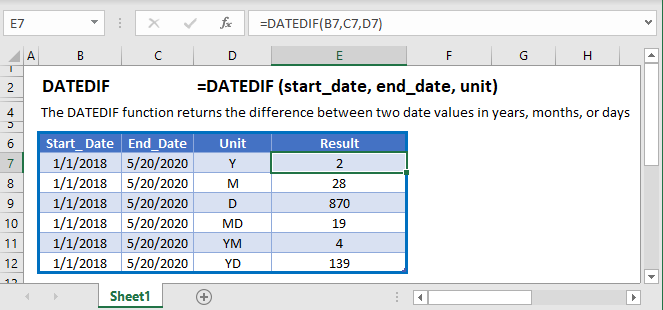## DATEDIF Function Overview

The DATEDIF Function Returns the number of days, months or years between two dates.

To use the DATEDIF Excel Worksheet Function, Select cell and Type:

(Unlike most Excel Functions, the DATEDIF inputs do not appear when you start typing the function)

## DATEDIF Function syntax and Inputs:

start_date – The start date in Excel serial number format. Example: You can not enter 11/12/2015 directly into the cell. Instead you would need to use the corresponding serial number: 42320. Alternatively, you can reference a cell with the date 11/12/2015 entered. Excel automatically converts dates stored in cells into serial format (unless the date is entered as text).

end_date – The end date in Excel serial number format. Example: You can not enter 11/12/2015 directly into the cell. Instead you would need to use the corresponding serial number: 42320. Alternatively, you can reference a cell with the date 11/12/2015 entered. Excel automatically converts dates stored in cells into serial format (unless the date is entered as text).

unit – The time unit to use. Enter “Y”

AutoMacro - VBA Code Generator

## DATEDIF Units

The DATEDIF Function can return the difference between two dates with various units: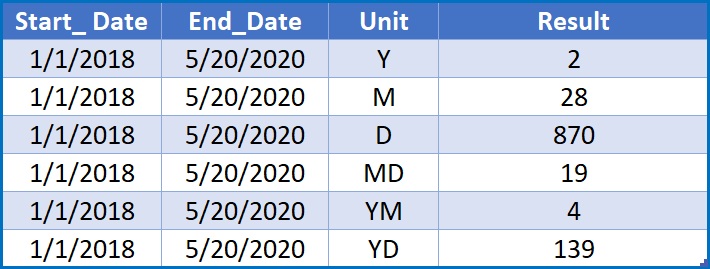Let’s look at some examples below.

## Count Days Between Dates

To count the numbers of days between dates you can use the DATEDIF Function: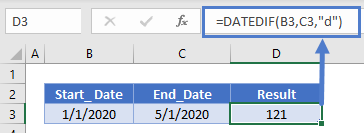You can also simply subtract the dates to find the difference in days between the dates.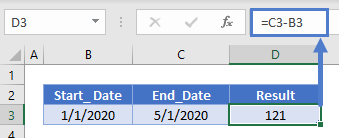<<Note for steve: link to article>>

Count Workdays Between Dates

To find the number of workdays between dates, use the NETWORKDAYS Function: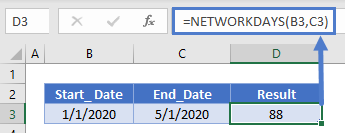Number Weeks Between Dates
Instead of days, you can count weeks by dividing dates by 07.

<<Note for steve: From Indika – “w” Not working so I am using Date/7 >>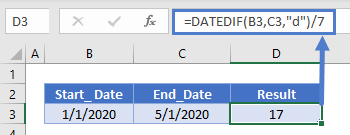## Date Difference in Months

Or months by setting the units to “m”: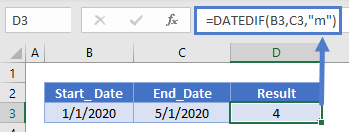Count Years Between Dates
Or years with “y”:

##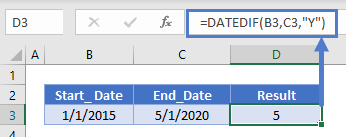DATEDIF in Google Sheets

The DATEDIF Function works exactly the same in Google Sheets as in Excel: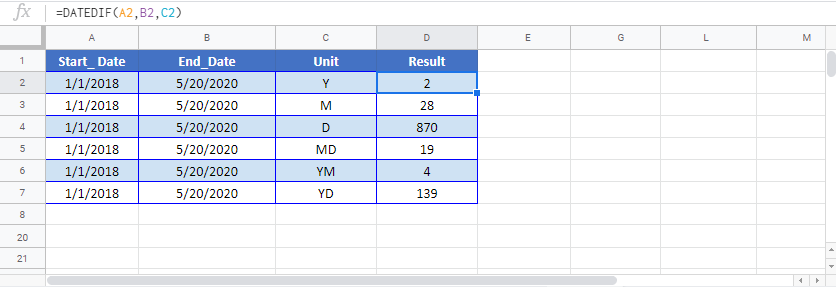## Excel Practice Worksheet

Practice Excel functions and formulas with our 100% free practice worksheets!

• Automatically Graded Exercises
• Learn Excel, Inside Excel!